prev next
 File: /Characters/UTF8/w.xml Author: MathML 2 Recommendation Description: Wide Characters starting with w Sample Rendering: N/A

Your browser's rendering:

namepicrender
Wcirc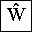$Ŵ$
wcirc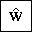$ŵ$
wedbar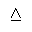$⩟$
Wedge$\bigwedge$
wedge$\wedge$
wedgeq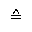$\stackrel{\wedge}{=}$
weierp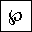$\wp$
Wfr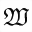$𝔚$
wfr$𝔴$
wopf$𝕨$
Wopf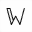$𝕎$
wp$\wp$
wr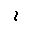$\wr$
wreath$\wr$
Wscr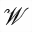$𝒲$
wscr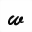$𝓌$

Source Code:

```        N/A
```